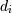## 9 th september, scatter plot example0

In the scatter plot example(9th september class), i did not understand what is the puspose of random generation of nos for colors and area?0

``````The main purpose of the scatter plot to understand how variables explained when we we perform multi variate analysis.
Like in the the plot variables x,y and z are identical.
When we perform x with y: the explanatory power has increased new insights where us to understand.``````0`numpy.random.``randn`(d0d1...dn)
If positive, int_like or int-convertible arguments are provided, `randn` generates an array of shape `(d0, d1, ..., dn)`, filled with random floats sampled from a univariate “normal” (Gaussian) distribution of mean 0 and variance 1 (if any of theare floats, they are first converted to integers by truncation).